### Example 7.2 Using the INQUAD= Option

This example illustrates the INQUAD= option for specifying a quadratic programming problem: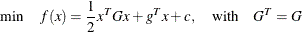Suppose that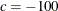,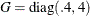and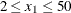,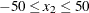, and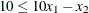.

You specify the constantand the Hessian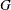in the data set QUAD1. Notice that the _TYPE_ variable contains the keywords that identify how the procedure should interpret the observations.

data quad1;
input _type_ $_name_$ x1 x2;
datalines;
const   .   -100  -100
;


You specify the QUAD1 data set with the INQUAD= option. Notice that the names of the variables in the QUAD1 data set and the _NAME_ variable match the names of the parameters in the PARMS statement.

proc nlp inquad=quad1 all;
min ;
parms x1 x2 = -1;
bounds  2 <= x1 <= 50,
-50 <= x2 <= 50;
lincon 10 <= 10 * x1 - x2;
run;


Alternatively, you can use a sparse format for specifying thematrix, eliminating the zeros. You use the special variables _ROW_, _COL_, and _VALUE_ to give the nonzero row and column names and value.

data quad2;
input _type_ $_row_$ _col_ $_value_; datalines; const . . -100 quad x1 x1 0.4 quad x2 x2 4 ;  You can also include the constraints in the QUAD data set. Notice how the _TYPE_ variable contains keywords that identify how the procedure is to interpret the values in each observation. data quad3; input _type_$ _name_ \$ x1   x2   _rhs_;
datalines;
const      .     -100  -100   .

proc nlp inquad=quad3;